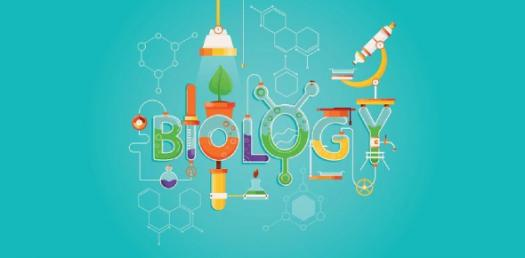# Biology Chapter 2 And 3 Quiz

8 Questions | Total Attempts: 426SettingsCh 2 and 3. Campbell Biology.

• 1.
Which of the following definitions is INCORRECT?
• A.

Potential Energy: Energy that matter possesses because of its location or structure

• B.

Element: Substance that cannot be broken down into other substances by chemical reactions.

• C.

Compound: Substance consisting of 2 or more different elements combined in a fixed ratio.

• D.

Trace Elements: Elements required by an organism in only minute quantities.

• E.

All of the above are true; none are incorrect.

• 2.
Which of the following definitions is CORRECT?
• A.

The number of electrons is unique to an element, thus defining it.

• B.

The 3-D space where an electron is found 90% of the time is called a shell.

• C.

The s orbital has a sphere shape, and the p orbital has a figure-8 shape.

• D.

Each s orbital can hold 2 electrons, while each p orbital can hold 6.

• E.

Covalent bonds and ionic bonds are weak chemical bonds.

• 3.
Which of the following can be used to represent a molecule?
• A.

Name (molecular formula)

• B.

Electron-shell diagram

• C.

Structural formula

• D.

Space filling model

• E.

All of the above

• 4.
Which of the following definitions is INCORRECT?
• A.

Cation: negatively charged ion.

• B.

Anion: positively charged ion

• C.

Ion: charged atom or molecule

• D.

A and B

• E.

None of the above are incorrect.

• 5.
Which of the following is INCORRECT?
• A.

Hydrogen bond: forms when a hydrogen atom covalently bonded to one electronegative atom is also attracted to an electropositive atom (eg. Oxygen, Nitrogen)

• B.

Van der Waals interactions are weak and only occur when atoms/molecules are very close together.

• C.

Cohesion: attraction between molecules of the same substance.

• D.

Polar molecule: opposite ends of the molecule have opposite charges. (eg. water is a polar molecule)

• E.

Adhesion: attraction between molecules of different substances.

• 6.
Which of the following is INCORRECT?
• A.

Atoms and molecules have kinetic energy because they are always randomly moving.

• B.

Ice is denser than water.

• C.

Aqueous solution: a solution where water is the solvent.

• D.

Solvent: dissolving agent of a solution.

• E.

Solute: substance that is dissolved.

• 7.
Which of the following is INCORRECT?
• A.

Hydrophobic = "water hating" & Hydrophilli =: "water loving"

• B.

Molar mass = Molecular Mass

• C.

1 mole = 6.02 x 10^23 "objects"

• D.

Molarity = number of moles of solute per liter of solution.

• E.

None of the above are incorrect.

• 8.
Which of the following is INCORRECT?
• A.

Hydrogen ion has a positive charge of +1

• B.

Hydroxide ion has a negative charge of -1

• C.

[H+][OH-} = 10^-14

• D.

PH = log [H+]

• E.

Pure water has a pH of 7, and is when [H+] = [OH-]Back to top